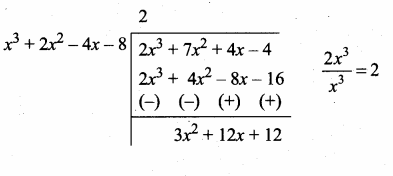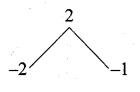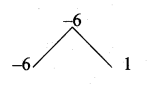## Ex 3.2 | Aster Classes

Question 1.
Find the GCD of the given polynomials by Division Algorithm
(i) x4 + 3x3 – x – 3, x3 + x2 – 5x + 3
p(x) = x4 + 3x3 – x – 3
g(x) = x3 + x2 – 5x + 3

3x2 + 6x – 9 = 3(x2 + 2x – 3)
Now dividing g(x) = x3 + x2 – 5x + 3
by the new remainder
(leaving the constant 3)
we get x2 + 2x – 3
G.C.F. = x2 + 2x – 3

(ii) x4 – 1, x3 – 11x2 + x – 11
p(x) = x4 – 1
g(x) = x3 – 11x2 + x – 11120x2 + 120 = 120 (x2 + 1)
Now dividing g(x) = x3 – 11x2 + x – 11 by the new remainder (leaving the constant) we get x2 + 1G.C.D. = x2 + 1

(iii) 3x4 + 6x3 – 12x2 – 24x, 4x4 + 14x3 + 8x2 – 8x
p(x) = 3x4 + 6x3 – 12x2 – 24x
= 3x (x3 + 2x2 – 4x – 8)
g(x) = 4x4 + 14x3 + 8x2 – 8x
= 2x (2x3 + 7x2 + 4x – 4)
G.C.D. of 3x and 2x = x
Now g(x) is divide by p(x) we get3x2 + 12x + 12 = 3 (x2 + 4x + 4)
Now dividing p(x) = x3 + 2x2 – 4x – 8
by the new remainder
(leaving the constant)
x2 + 4x + 4G.C.D. = x(x2 + 4x + 4) [Note x is common for p(x) and g(x)]

(iv) 3x3 + 3x2 + 3x + 3, 6x3 + 12x2 + 6x+12
p(x) = 3x3 + 3x2 + 3x + 3
= 3(x3 + x2 + x + 1)
g(x) = 6x3 + 12x2 + 6x + 12
= 6(x3 + 2x2 + x + 2)
G.C.D. of 3 and 6 = 3
Now g(x) is divided by p(x)

Now dividing p(x) by the remainder x2 + 1
we get x + 1

∴ G.C.D. = 3(x2 + 1) [3 is the G.C.D. of 3 and 6]

Question 2.
Find the LCM of the given polynomials
(i) 4x2y, 8x3y2
4x2 y = 2 × 2 × x2 × y
8 x3 y2 = 2 × 2 × 2 × x3 × y2
L.C.M. = 23 × x3 × y2
= 8x3y2

Aliter: L.C.M of 4 and 8 = 8
L.C.M. of x2y and x3y2 = x3y2
∴ L.C.M. = 8x3y2

(ii) -9a3b2, 12a2b2c
-9a3b2 = -(32 × a3 × b2)
12a2b2c = 22 × 3 × a2 × b2 × c
L.C.M. = -(22 × 32 × a3 × b2 × c)
= -36 a3b2c

(iii) 16m, -12m2n2, 8n2
16m = 24 × m
-12 m2n2 = -(22 × 3 × m2 × n2)
8n2 = 23 × n2
L.C.M. = -(24 × 3 × m2 × n2)
= -48 m2n2

(iv) p2 – 3p + 2, p2 – 4
P2 – 3p + 2 = p2 – 2p – p + 2
= p(p – 2) – 1 (p – 2)
= (p – 2) (p – 1)p2 – 4 = p2 – 22 (using a2 – b2 = (a + b) (a – b)]
= (p + 2) (p – 2)
L.C.M. = (p – 2) (p + 2) (p – 1)

(v) 2x2 – 5x – 3,4.x2 – 36
2x2 – 5x – 3 = 2x2 – 6x + x – 3
= 2x (x – 3) + 1 (x – 3)
= (x – 3) (2x + 1)= 4x2 – 36 = 4 [x2 – 9]
= 4 [x2 – 32]
= 4(x + 3) (x – 3)
L.C.M. = 4(x – 3) (x + 3) (2x + 1)

(vi) (2x2 – 3xy)2,(4x – 6y)3,(8x3 – 27y3)
(2x2 – 3xy)2 = x2 (2x – 3y)2
(4x – 6y)3 = 23 (2x – 3y)3
= 8 (2x – 3y)3
8x3 – 27y3 = (2x)3 – (3y)3
= (2x – 3y) [(2x)2 + 2x × 3y + (3y2)]
[using a3 – b3 = (a – b) (a2 + ab + b2)
(2x – 3y) (4x2 + 6xy + 9y2)
L.C.M. = 8x2 (2x – 3y)3 (4x2 + 6xy + 9y)2

#### VISITORS COUNTUsers Today : 198Total Users : 166829Views Today : 414Total views : 617227